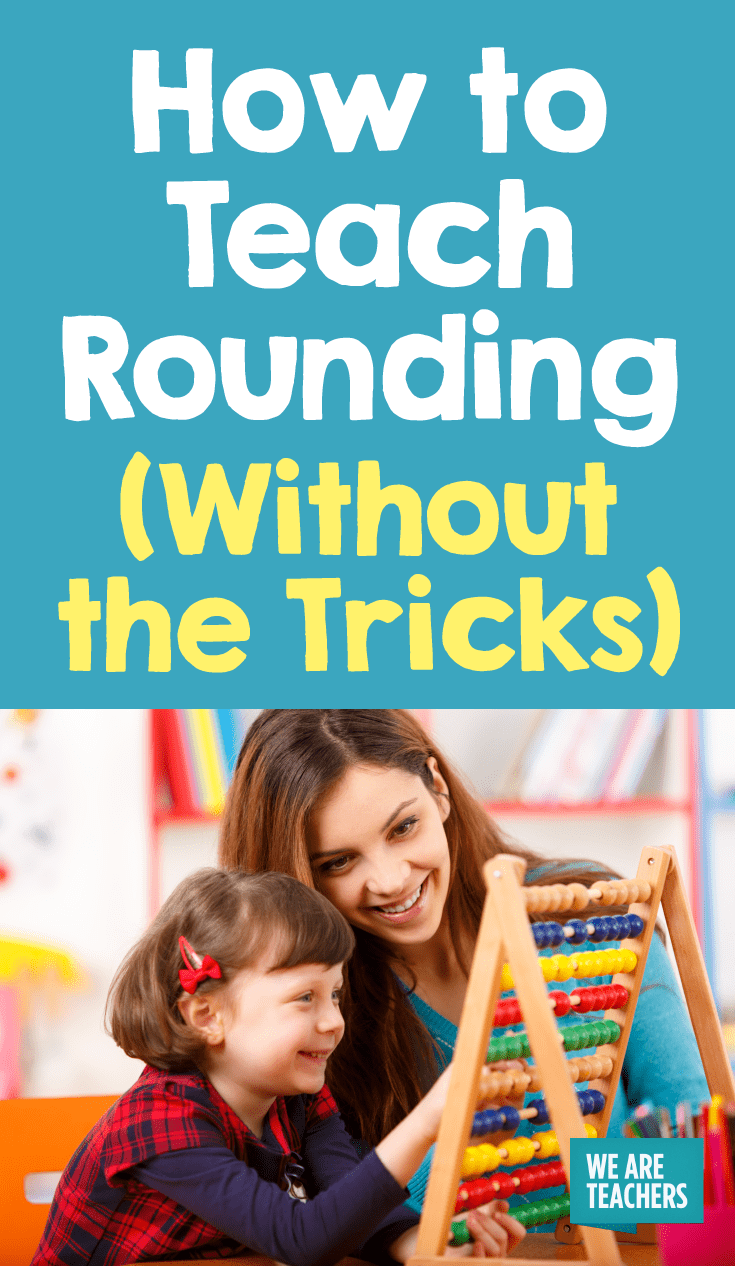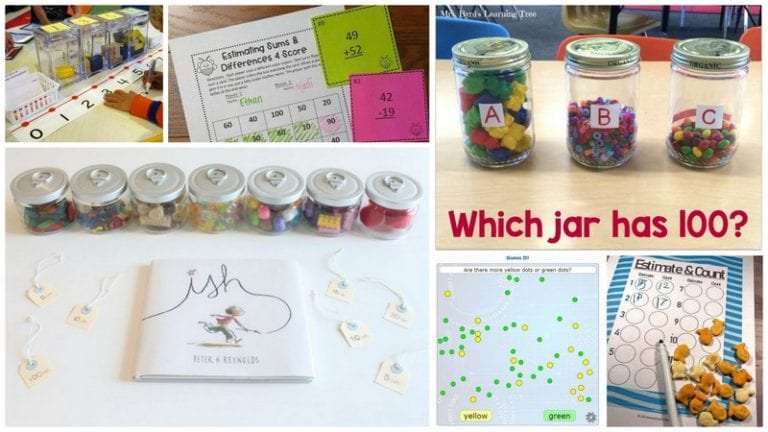# Rounding “Tricks” Miss the Point of Teaching Rounding

Even if your students are getting the right answer, it isn’t always enough.

I recently watched a third grade student fly through a problem set that asked her to round two-digit numbers to the nearest ten. She rounded 83 to 80 and 27 to 30 with ease.

But when asked to round 283 to the nearest ten, she wrote 300. 1849 rounded to the nearest hundred was also challenge. She was circling and underlining like crazy, but it didn’t seem to help when the problems increased in difficulty.

She then launched into a lengthy explanation: “If it’s above five you round up, and if it is below five you round down.” While technically true, she didn’t know why five was the cut-off point for rounding up. She also made no mention of place value.

So she (sorta) knew how to round without actually knowing what rounding is. Deep breath.

## Why Getting the Right Answer When Rounding Isn’t Enough

Like the “alligator mouth” for the greater than and less than symbols, we often teach rounding in a way that yields the correct answer but not an increased understanding of place value.

Any trick’s major shortcoming is that students struggle to apply it when faced with different kinds of problems. My student could round two-digit numbers to the nearest ten, but that was the only way she knew how to apply the steps she’d learned.

The trick didn’t give her the know-how to apply it flexibly because she didn’t understand why she was taking the steps she was taking.

Rounding is a key way for students to learn about place value, but tricks often take the place value practice out of it.

## Instead, Model Rounding on an Open Number Line

The reason rounding “tricks” are so prevalent is that they offer the hope that rounding can be taught as a series of steps, separate from other math concepts. Without developing a true understanding of place value, though, rounding will only ever be steps.

Start with rounding two-digit numbers using an open number line model. I like to ask what tens number comes before and after the number being rounded. These two tens numbers create the two endpoints of our number line.

For 83 rounded to the nearest ten, the number line would have 80 at one end and 90 at the other end. Then place 85 in the middle, pointing out that 85 is right in the middle between 80 and 90. These steps might seem basic, but this is the necessary work of grasping place value.

Next, ask students to place the number being rounded onto the number line. For some students, this might be challenging. But if they can’t accurately place 83 between 80, 85 and 90, then you can see why rounding is an important concept to continue to practice.

Finally, ask students if the number they just plotted—83 in our example—is closer to 80 or 90, the two closest tens.

When students learn to round by placing the number in question on an open number line, they have a visual model to support their understanding of both rounding and place value.## You Might Also Like